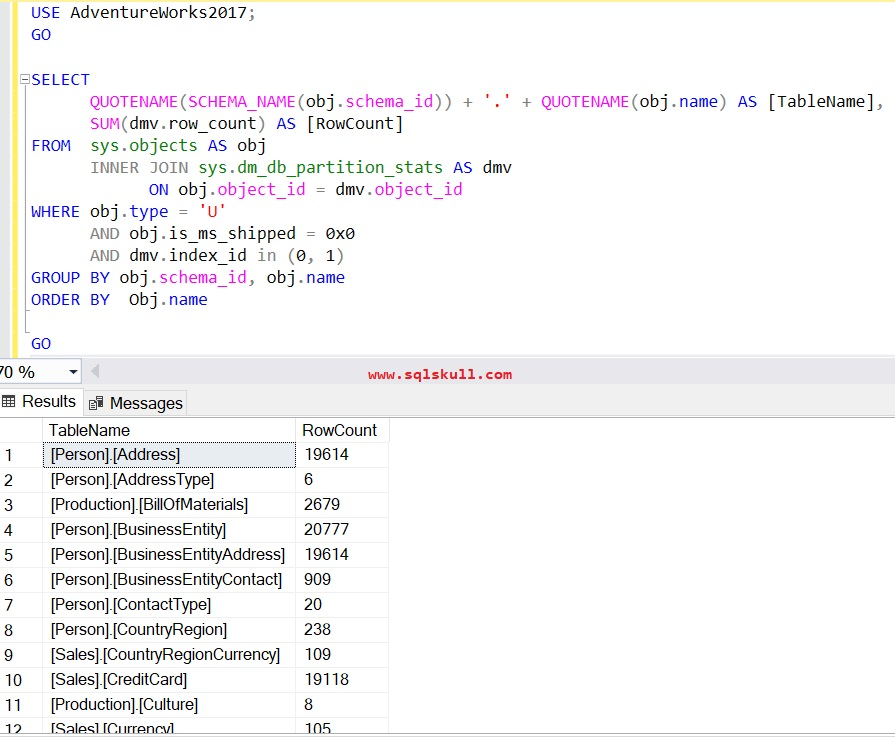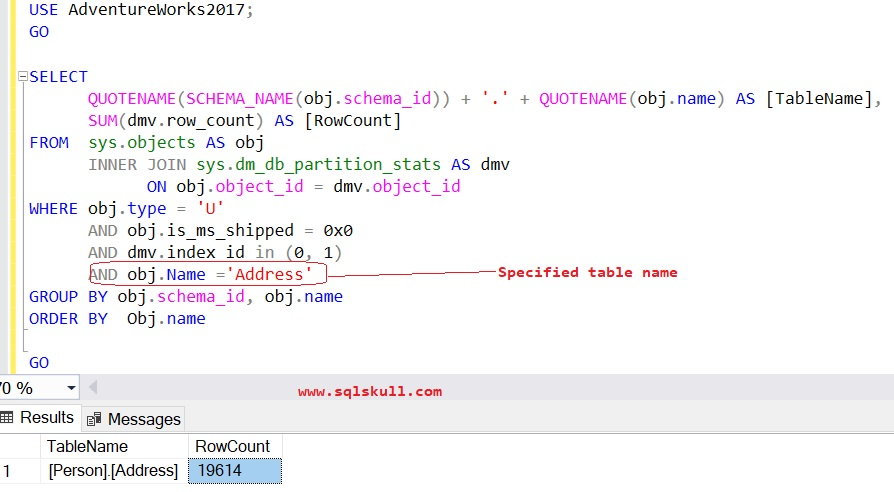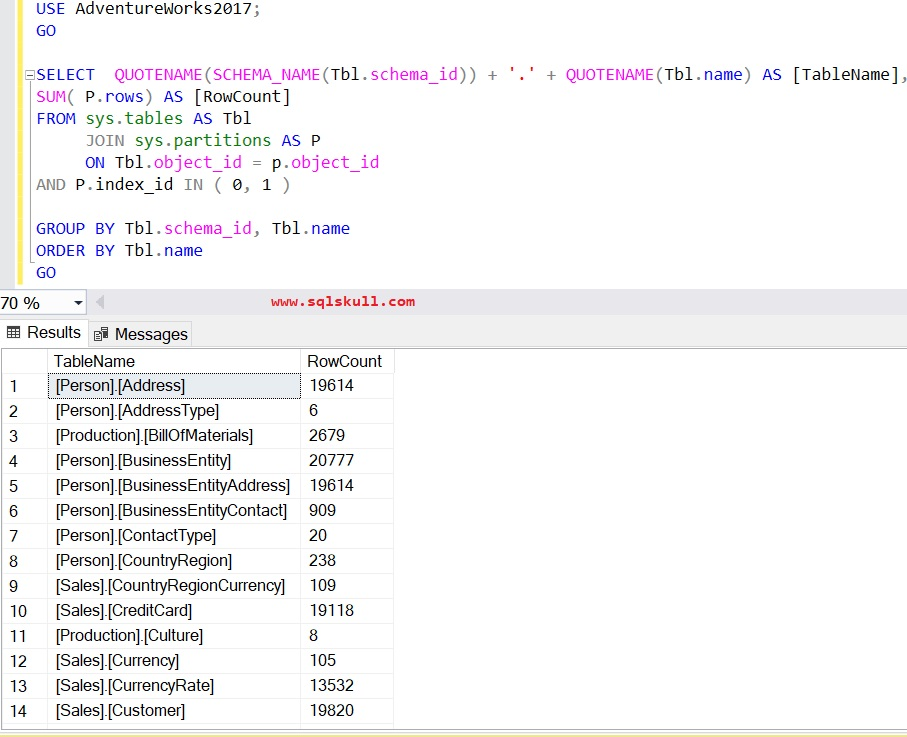Home » Get Row Counts for all tables in SQL Server

# Get Row Counts for all tables in SQL ServerSometime you are required to validate the row counts for each tables in database. So getting a row counts for each tables either through using a loop on each table or go and get row counts one by one for each table in database, definitely that will be a lengthy process and take a lot of your time.

In this situation, the best way would be using any SQL Server scripts which provides a row counts information of tables.

In SQL Server there some System Dynamic Management View, and System catalog views that you can use for getting a row counts for each tables in SQL Server database.

### Row counts for each tables using sys.dm_db_partition_stats

dm_db_partition_stats is a SQL Server dynamic management view which returns a page and row-count information for each partition in the current database.

Following T-SQL uses dmv dm_db_partition_stats and returns row counts for each tables in Database AdventureWorks2017.

```USE AdventureWorks2017;
GO

SELECT
QUOTENAME(SCHEMA_NAME(obj.schema_id)) + '.' + QUOTENAME(obj.name) AS [TableName],
SUM(dmv.row_count) AS [RowCount]
FROM sys.objects AS obj
INNER JOIN sys.dm_db_partition_stats AS dmv
ON obj.object_id = dmv.object_id
WHERE obj.type = 'U'
AND obj.is_ms_shipped = 0x0
AND dmv.index_id in (0, 1)
GROUP BY obj.schema_id, obj.name
ORDER BY Obj.name

GO```You can see, it returns row counts for each table in database Adventureworks2017.

In case, if you want to see row counts for specific table then you can specify a table name in where condition as shown below.

```USE AdventureWorks2017;
GO

SELECT
QUOTENAME(SCHEMA_NAME(obj.schema_id)) + '.' + QUOTENAME(obj.name) AS [TableName],
SUM(dmv.row_count) AS [RowCount]
FROM sys.objects AS obj
INNER JOIN sys.dm_db_partition_stats AS dmv
ON obj.object_id = dmv.object_id
WHERE obj.type = 'U'
AND obj.is_ms_shipped = 0x0
AND dmv.index_id in (0, 1)
GROUP BY obj.schema_id, obj.name
ORDER BY Obj.name

GO```### Row counts for each tables using sys.partitions

sys.partitions is a SQL Server System Catalog view which contains a row for each partition of all the tables and most types of indexes in the database. Using this you can get a row counts for each tables in database.

```USE AdventureWorks2017;
GO

SELECT
QUOTENAME(SCHEMA_NAME(Tbl.schema_id)) + '.' + QUOTENAME(Tbl.name) AS [TableName],
SUM( P.rows) AS [RowCount]
FROM sys.tables AS Tbl
JOIN sys.partitions AS P
ON Tbl.object_id = p.object_id
AND P.index_id IN ( 0, 1 )

GROUP BY Tbl.schema_id, Tbl.name
ORDER BY Tbl.name

GO```You can see, it returns row counts for each table in database Adventureworks2017.